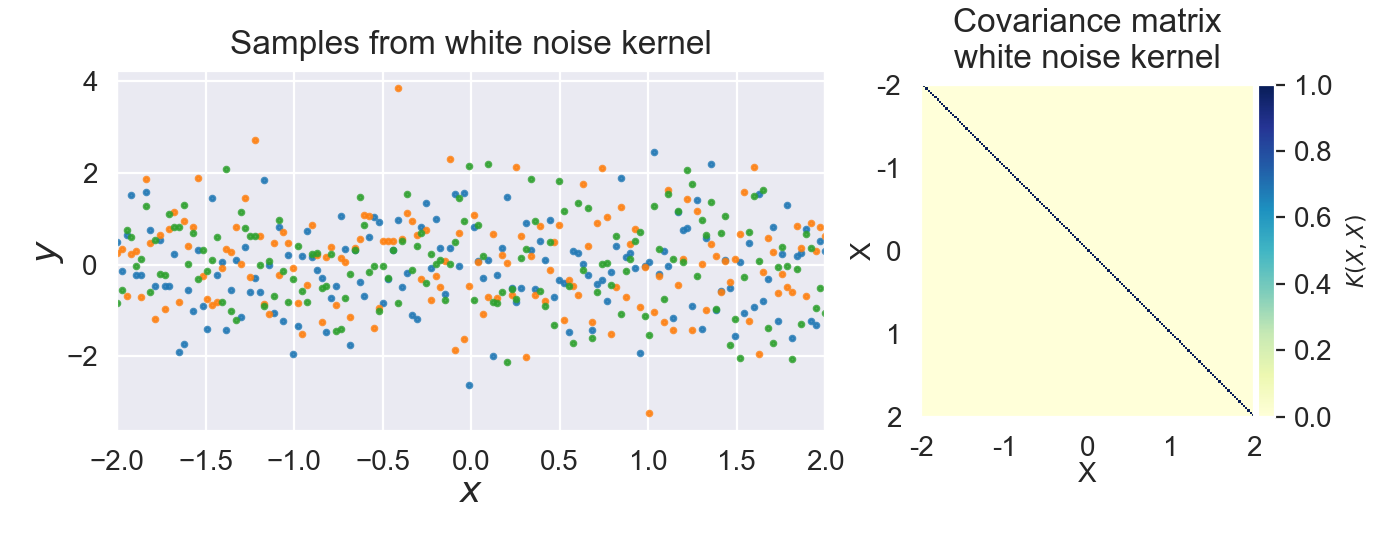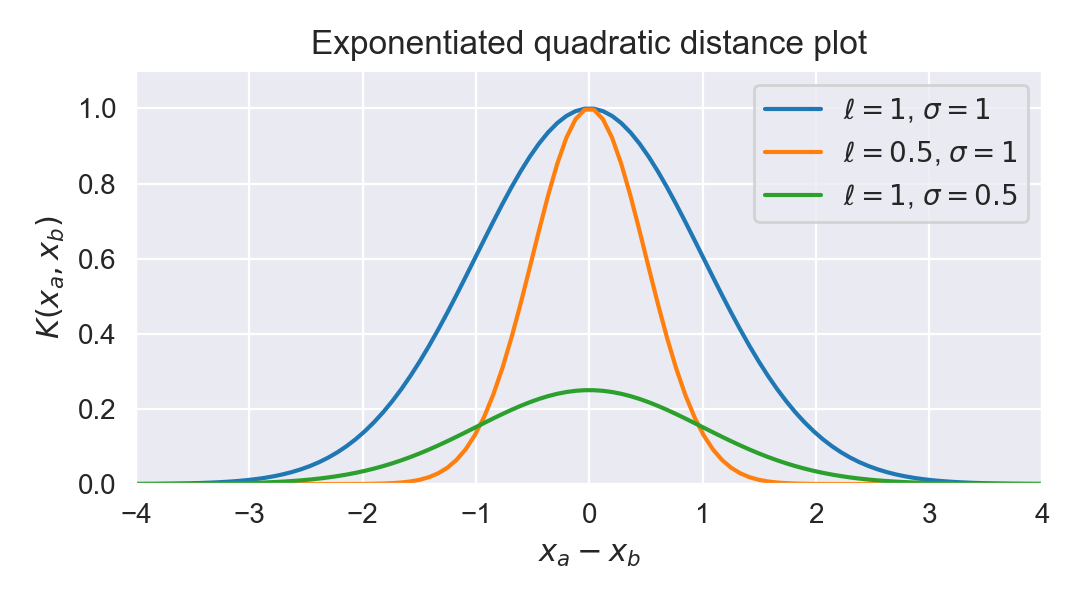# Gaussian process kernels

This post will go more in-depth in the kernels fitted in our example fitting a Gaussian process to model atmospheric CO₂ concentrations . We will describe and visually explore each part of the kernel used in our fitted model, which is a combination of the exponentiated quadratic kernel, exponentiated sine squared kernel, and rational quadratic kernel. This post is part of series on Gaussian processes:

In :

## Kernel function ¶

A kernel (or covariance function) describes the covariance of the Gaussian process random variables. Together with the mean function the kernel completely defines a Gaussian process.

In the first post we introduced the concept of the kernel which defines a prior on the Gaussian process distribution. To summarize the kernel function $k(x, x')$ models the covariance between each pair in $x$. The kernel function together with the mean function $m(x)$ define the Gaussian process distribution:

$$y \sim \mathcal{GP}(m(x),k(x,x'))$$

### Valid kernels ¶

In order to be a valid kernel function the resulting kernel matrix $\Sigma = k(X, X)$ should be positive definite . Which implies that the matrix should be symmetric . Being positive definite also means that the kernel matrix is invertible .

The process of defining a new valid kernel from scratch it not always trivial. Typically pre-defined kernels are used to model a variety of processes. In what follows we will visually explore some of these pre-defined kernels that we used in our fitting example .

In :

## White noise kernel ¶

The white noise kernel represents independent and identically distributed noise added to the Gaussian process distribution.

$$k(x, x) = \sigma^2 I_n$$

With:

• $\sigma^2$ the variance of the noise.
• $I_n$ the identity matrix.

This formula results in a covariance matrix with zeros everywhere except on the diagonal of the covariance matrix. This diagonal contains the variances of the individual random variables. All covariances between samples are zero because the noise is uncorrelated.

Samples from the white noise kernel together with a visual representation of the covariance matrix are plotted in the next figure.

In :The exponentiated quadratic kernel (also known as squared exponential kernel, Gaussian kernel or radial basis function kernel) is one of the most popular kernels used in Gaussian process modelling. It can be computed as:

$$k(x_a, x_b) = \sigma^2 \exp \left(-\frac{ \left\Vert x_a - x_b \right\Vert^2}{2\ell^2}\right)$$

With:

• $\sigma^2$ the overall variance ($\sigma$ is also known as amplitude).
• $\ell$ the lengthscale.

Using the exponentiated quadratic kernel will result in a smooth prior on functions sampled from the Gaussian process.

In :
def exponentiated_quadratic_tf(amplitude, length_scale):
amplitude_tf = tf.constant(amplitude, dtype=tf.float64)
length_scale_tf = tf.constant(length_scale, dtype=tf.float64)
amplitude=amplitude_tf,
length_scale=length_scale_tf)
return kernel

kernel_matrix = kernel.matrix(xa, xb)
with tf.Session() as sess:
return sess.run(kernel_matrix)


The exponentiated quadratic is vizualized in the next figures. The first figure shows the distance plot with respect to $0$: $k(0, x)$. Note that the similarity outputted by the kernel decreases exponentially towards $0$ the farther we move move away from the center, and that the similarity is maximum at the center $x_a = x_b$.

In :The following figure shows samples from the exponentiated quadratic kernel together with a visual representation of its covariance matrix.

Observe in the previous and following figure that increasing the lengthscale parameter $\ell$ increases the spread of the covariance. Increasing the amplitude parameter $\sigma$ increases the maximum value of the covariance.

In :